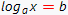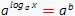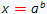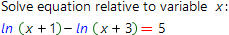# Logarithmic equations online calculatorLogarithmic is called an equation where unknown variable is the argument of logarithm function, for example:To solve such equations one need to use the logarithm features:From the equation above, find the variable x:The equation above is the simplest one. It is possible that in more complicated cases one need to use another logarithm features. Our calculator, build on Wolfram Alpha system is able to solve any, even very complicated logarithmic equations with step by step solution.

Logarithmic equations calculatorLogarithmic equation you want to solve: